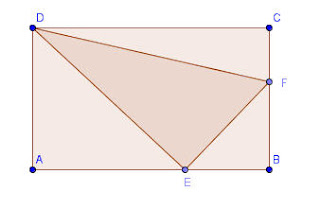## Monday, 19 October 2009

In a recent blog, I asked what I thought was An Interesting Geometry Problem. Several emails and a comment later, I thought it was time to give a solution.

Before I do, I want to gloat. I gave the problem to some of my students in seminar today, which includes a couple of bright young students, and in a matter of moments, Tyler P. and his Ti-89 solved it. He began by assuming "If it is true for any rectangle, it is true for a square." and worked from there. I don't know if that really makes the solution simpler, but it is the type of problem solving that marks a budding mathematician. He got the solution, but didn't recognize it as a special number, although when I told him what it was, he had heard of the name... note to self, give my students more exposure to recreational math topics.So here is my solution, hope it is correct.
For ease of notation, I will refer to the lengths AE as x, EB as y, so that CD=x+y ... and similarly I will let BF=s and FC=t so that AD=s+t

Since the three triangles ADE, EBF, and FCD are all equal in area, it must be true that (s+t)x = ys=(x+y)t.

Expanding the first and last of these we get sx+tx=xt+yt and so sx=yt if we divide by xt we reveal the fact that s/t=y/x and so we see that the ratios of the partitions are equal on each side with corresponding parts adjacent to vertex B.

If instead, we divide sx=yt by only the t we have y=sx/t.

Now using that, we can substitute into the equality (s+t)x= ys to get sx + tx = s^2 x/t.

Factoring out an x, since it can not be zero, we get s + t = s^2/t and now if we divide all tems by t we get a quadratic in the ratio (s/t)... s/t + 1 = (s/t)^2 but this is quickly recognized to yield the conjugate of the divine proportion, ...Solving by any quadratic method we get s/t =so we see that the three triangles are all equal when the lengths AB and BC are divided in the golden ratio with the larger section adjacent to B.

If we find the area of one of these triangles, and compare it to the area of DEF, we derive a, perhaps unexpected, result that it is impossible to inscribe a triangle in a rectangle and have all four triangles have equal area.

Interestingly, the ratio of the area of DEF to the other triangles isor appx 2.23606797

Mary O'Keeffe said...

This was indeed a lovely problem. I solved it the same way as your student did, so of course I thought his insight was very elegant!

It's simpler to work with a square, because it reduces the number of parameters you have to carry around in your algebra.

It's easy to see that you can take any given arbitrary rectangle meeting the specs of the original problem and transform it into a square by a suitable rescaling of the units in a way that preserves the equality of the three triangles, so his method clearly generalizes beyond squares.Anonymous said...

I have a question when u did the area, isn't the area of the triangle A= 1/2bh, i didn't see any 1/2. Confused.

Pat's Blog said...

J kid,
But if two trangles are equal, then twice the area of one is still equal to twice the area of hte other... ie if 1/2 AB = 1/2 CD, then AB = CD... that was what happened, and since I wasn't actually looking for the areas, but just the partition that made them equal, I could ignore the 1/2. Hope that's clear...